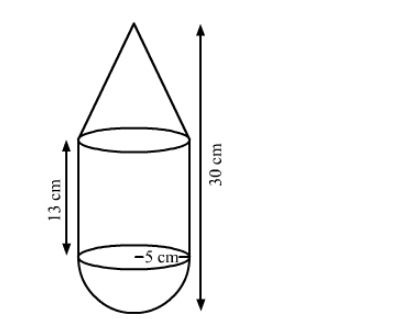# A toy is in the shape of a right circular cylinder with a hemisphere on one end and a cone on the other.`
Question:

A toy is in the shape of a right circular cylinder with a hemisphere on one end and a cone on the other. The radius and height of the cylindrical part are 5 cm and 13 cm, respectively. The radii of the hemispherical and the conical parts are the same as that of the cylindrical part. Find the surface area of the toy, if the total height of the toy is 30 cm.

Solution:We have,

the base radius of cone $=$ the base radius of cylinder $=$ the base radius of hemisphere $=r=5 \mathrm{~cm}$,

the height of the cylinder, $H=13 \mathrm{~cm}$,

the total height of the toy $=30 \mathrm{~cm}$

Also, the height of the cone, $h=30-(13+5)=12 \mathrm{~cm}$

The slant heigt of the cone, $l=\sqrt{r^{2}+h^{2}}$

$=\sqrt{5^{2}+12^{2}}$

$=\sqrt{25+144}$

$=\sqrt{169}$

$=13 \mathrm{~cm}$

Now, the surface area of the toy $=$ CSA of cone + CSA of cylinder + CSA of hemisphere

$=\pi r l+2 \pi r H+2 \pi r^{2}$

$=\pi r(l+2 H+2 r)$

$=\frac{22}{7} \times 5 \times(13+2 \times 13+2 \times 5)$

$=\frac{22}{7} \times 5 \times(13+26+10)$

$=\frac{22}{7} \times 5 \times 49$

$=770 \mathrm{~cm}^{2}$

So, the surface area of the toy is $770 \mathrm{~cm}^{2}$.